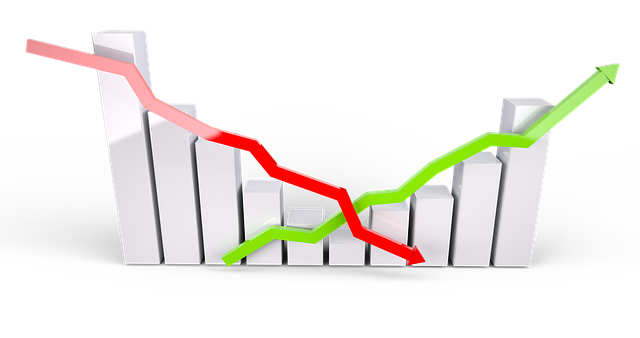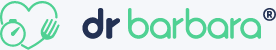Digital Tbucket Tank (DTT)

# Mathematics for EconomistsMathematical economics is the application of mathematical methods to present theories and to analyze problems in economics. Often these applied methods go beyond simple geometry and may include differential and integral calculus, differential and differential equations, matrix algebra, mathematical programming, or other computational methods.
Mathematics enables economists to make meaningful, testable statements about wide-ranging and complex issues that are less easily expressed through informal channels. In addition, the language of mathematics enables economists to make specific, positive claims about controversial or contentious issues that would not be possible without mathematics.

Focus:

Linear Algebra

Analysis

Differential equations

### Customers and Partners